Function Repository Resource:

# RationalFunctionQ

Determine whether an expression represents a rational function of a given set of variables

Contributed by: Wolfram Staff
 ResourceFunction["RationalFunctionQ"][expr,var] returns True if expr is a rational function of the symbol var, and returns False otherwise. ResourceFunction["RationalFunctionQ"][expr,{var1,var2, …}] returns True if expr is a rational function of each of the symbols vari and returns False otherwise.

## Examples

### Basic Examples (5)

Test whether an expression represents a rational function of a given variable:

 In:=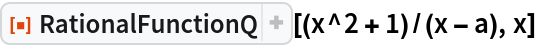Out=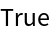Test a different expression:

 In:=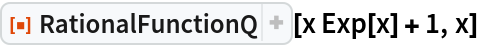Out=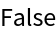Test for rationality with respect to a list of variables:

 In:=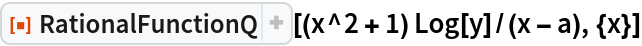Out=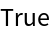Test another expression for rationality with respect to a list of variables:

 In:=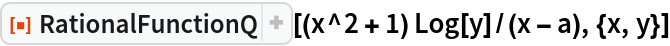Out=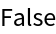Test another expression for rationality with respect to a list of variables:

 In:=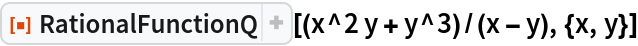Out=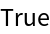### Possible Issues (1)

Expressions which are constant with respect to a variable are considered rational:

 In:=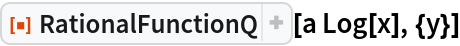Out=In:=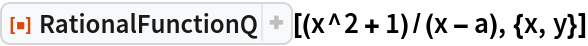Out=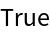## Version History

• 2.0.0 – 10 July 2019
• 1.0.0 – 05 March 2019